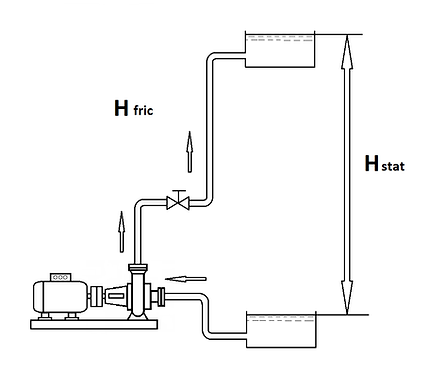## PUMPING SYSTEM CURVE

###### What is necessary to know about the piping system characteristic for the correct pump selection?

The clear understanding of systems characteristic is the necessary condition for the right pump selection and efficient pump operation.

The system offers resistance to the flow in two ways that are fundamentally different. The work to lift anything is mgh where m is the mass lifted, g is the gravitational constant and h is how high the mass was lifted. The pump sometimes lifts fluid and it always has to overcome frictional forces. These two types of work are fundamentally different and has to be dealt with in different ways. The pressure to overcome when lifting the fluid equals the pressure difference between the pump and the outlet from the system with zero flow. It is the pressure that would be measured at the pump using a pressure gauge or the height of the fluid at the outlet minus the height where the pump is.

The piping system characteristic has two parts - static head and friction losses.Pressure Resistance of a System

• It takes energy to lift fluid from one level to another

• The pressure used to lift fluid is called Static Head

• The energy used to lift fluid is independent of velocity

• The power to lift fluid is a linear function of velocity

• It takes energy to move fluid through a system of pipes and other equipment.

• The pressure used to overcome friction is called the or Dynamic Friction Head.

• The pressure required is proportional to the square of the fluid velocity

• The power is proportional to the cube of the velocity

Static head Hstat includes geometrical height where the liquid has to be lifted and differences in pressures above liquid surfaces in intake reservoir and pressure reservoir.

Friction losses include friction losses in pipes losses in local resistances valves, pipe bends, diffusers, etc all places where the flow changes its shape.

Friction losses are described by the formula

###### Pipe friction loss estimates are based on an equation referred to as Darcy-WeisbachThis equation is very useful to examine to understand what parameters influence frictional losses in piping:

Hf - pressure drop due to friction (ft or m)

f - Darcy friction factor

L - pipe length (ft or m)

d - pipe diameter (ft or m)

V - velocity head (ft or m)where

k -  coefficient which depends on the element characteristic.

Q - is a flow goes through the elements

- Function of fluid velocity

- Lower flow (lower velocity) results in lower head loss

- Head loss is proportional to the square of velocity

- Reduced to 25% when velocity is cut in half !

- Increases by a factor of 4 when velocity is doubled

Depending on what share the static head and friction losses have in overall the system curve systems can be separated on systems with preliminary static head and preliminary friction losses.Pump System curve.###### How to build the system curve?

The graphical presentation is the most obvious way to understand the characteristics of a piping system and the influence of every element on the overall characteristic.

###### Step 1.

Draw curves of each element of a piping system, valves, pipes connections, pipe bends, etc.

###### Step 2.

If elements are connected in series the same flow goes through every element. It means that for building a system curve it is necessary to add the head of every element at the same flows.###### Step 3.

If a piping system has parallel branches the flow is divided but the values of pressure at the common points will be the same.

In this case, the summarized system characteristic will be received by adding characteristics of branches at the same head.

###### Step 4.

Draw the summarized curve of a pumping system.

###### The operating point of a pump is the intersection of a pump curve and a system curve.